Next: Waveguides Up: Resonant Cavities and Waveguides Previous: Axially Symmetric Cavities

# Cylindrical Cavities

Let us apply the methods of the previous section to the TM modes of a right circular cylinder of radius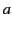. We can write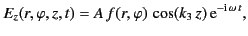(1344)

where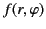satisfies the equation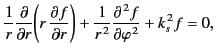(1345)

and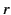,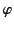,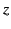are cylindrical coordinates. Let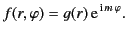(1346)

It follows that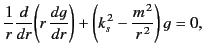(1347)

or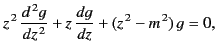(1348)

where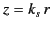. The above equation can be recognized as Bessel's equation. The independent solutions of this equation are denoted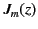and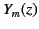. Theare regular at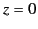, whereas theare singular. Moreover, both solutions are regular at large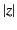.

Because the axis (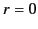) lies within the cavity, the radial eigenfunction must be regular at. This immediately rules out the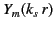solutions. Thus, the most general solution for a TM mode is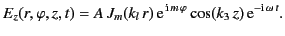(1349)

The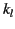are the eigenvalues of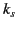, and are determined by the solution of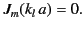(1350)

The above constraint ensures that the tangential electric field is zero on the conducting walls surrounding the cavity (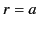).

The most general solution for a TE mode is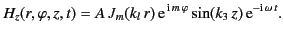(1351)

In this case, theare determined by the solution of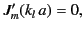(1352)

where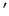denotes differentiation with respect to argument. The above constraint ensures that the normal magnetic field is zero on the conducting walls surrounding the cavity. The oscillation frequencies of both TM and TE modes are given by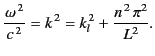(1353)

If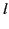is the ordinal number of a zero of a particular Bessel function of order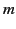(defined such thatincreases with increasing values of the argument) then each mode is characterized by three integers,,,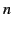, as in the rectangular case. Theth zero ofis conventionally denoted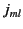[so,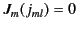]. Likewise, theth zero of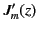is denoted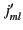. Table 2 shows the first few zeros of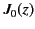,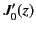,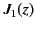, and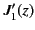. It is clear that, for fixedand, the lowest frequency mode (i.e., the mode with the lowest value of) is a TE mode. The mode with the next highest frequency is a TM mode. The next highest frequency mode is a TE mode, and so on.

Table: The first few values of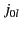,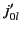,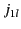and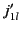.1 2.4048 0.0000 3.8317 1.8412 2 5.5201 3.8317 7.0156 5.3314 3 8.6537 7.0156 10.173 8.5363 4 11.792 10.173 13.324 11.706Next: Waveguides Up: Resonant Cavities and Waveguides Previous: Axially Symmetric Cavities
Richard Fitzpatrick 2014-06-27